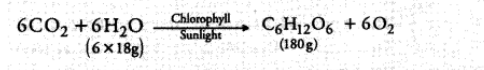# In photosynthesis, 6 molecules of carbon dioxide`
Question:

In photosynthesis, 6 molecules of carbon dioxide combine with an equal number of water molecules through a complex sereies of reactions to give a molecule of glucose having a molecular formula $\mathrm{C}_{6} \mathrm{H}_{12} \mathrm{O}_{6}$ and a molecule of oxygen with molecular formula $\mathrm{O}_{2}$. How many grams of water would be required to produce $18 \mathrm{~g}$ of glucose? Compute the volume of water so consumed assuming the density of water to be $1 \mathrm{~g} \mathrm{~cm}^{-3}$.

Solution:

The chemical reaction involved is:To produce $180 \mathrm{~g}$ of glucose, mass of water consumed $=108 \mathrm{~g}$

To produce $18 \mathrm{~g}$ of glucose, mass of water consumed $=\frac{(108 \mathrm{~g})}{(180 \mathrm{~g})} \times(18 \mathrm{~g})=10.8 \mathrm{~g}$

Density of water $=1 \mathrm{~g} \mathrm{~cm}^{-3}$

Volume of water $=\frac{\text { Mass of water }}{\text { Density of water }}=\frac{(10.8 \mathrm{~g})}{\left(1 \mathrm{~g} \mathrm{~cm}^{-3}\right)}=10.8 \mathrm{~cm}^{3} .$